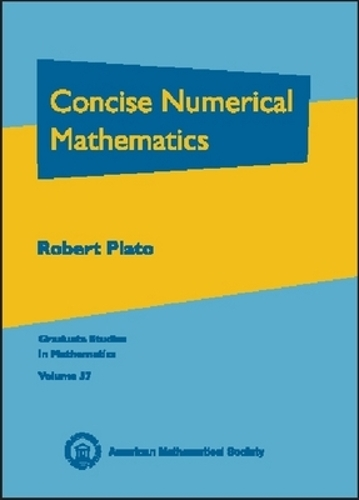•# Concise Numerical Mathematics - Graduate Studies in Mathematics (Hardback)

(author)
£97.95
Hardback Published: 30/05/2003
• We can order this

Usually dispatched within 3 weeks

This book succinctly covers the key topics of numerical methods. While it is basically a survey of the subject, it has enough depth for the student to walk away with the ability to implement the methods by writing computer programs or by applying them to problems in physics or engineering. The author manages to cover the essentials while avoiding redundancies and using well-chosen examples and exercises. The exposition is supplemented by numerous figures. Work estimates and pseudo codes are provided for many algorithms, which can be easily converted to computer programs. Topics covered include interpolation, the fast Fourier transform, iterative methods for solving systems of linear and nonlinear equations, numerical methods for solving ODEs, numerical methods for matrix eigenvalue problems, approximation theory, and computer arithmetic.In general, the author assumes only a knowledge of calculus and linear algebra. The book is suitable as a text for a first course in numerical methods for mathematics students or students in neighboring fields, such as engineering, physics, and computer science.

Publisher: American Mathematical Society
ISBN: 9780821829530
Weight: 1021 g